# Kerala Plus Two Maths Previous Question Papers and Answers 2018 In PDF

## Kerala Class 12th Maths Question Paper 2018 Solved – Free Download

Kerala Plus two (Class 12) maths 2018 question paper with answers are provided here in a downloadable pdf format and also in the text, so that the students can efficiently face the exams. Along with the solutions, Kerala Plus two maths question paper 2018 is also added here for better preparation. 12th Students are able to access all the plus two previous year maths question papers. The solution provided by BYJU’S will assist the students and help them verify their answers. It can be downloaded easily and in a shorter period of time. Solving 2018 Maths question papers and also the previous year papers for Class 12 is necessary and important in your preparation for the board exams.

### KBPE Plus Two Class 12th Maths Question Paper With Solution 2018

#### QUESTION PAPER CODE 9018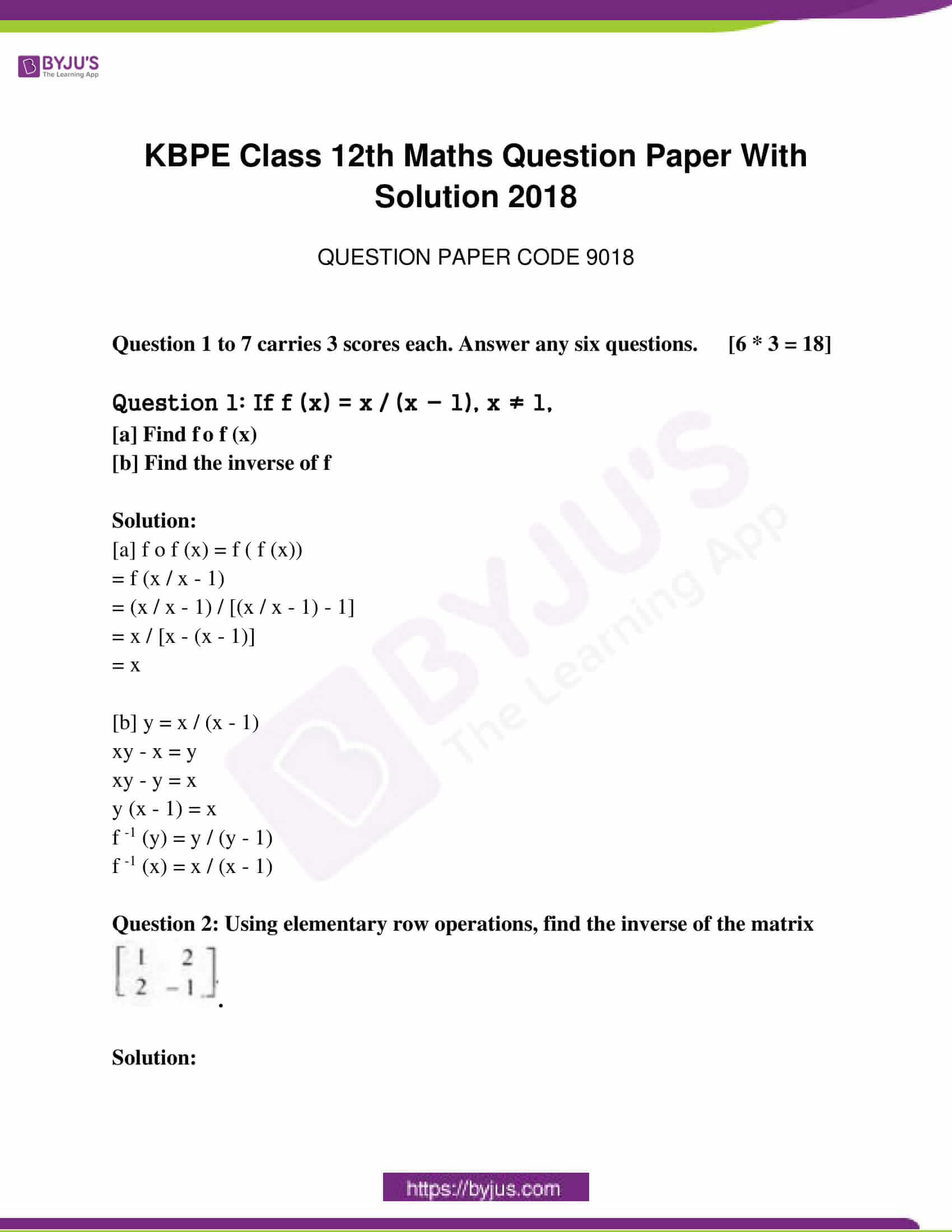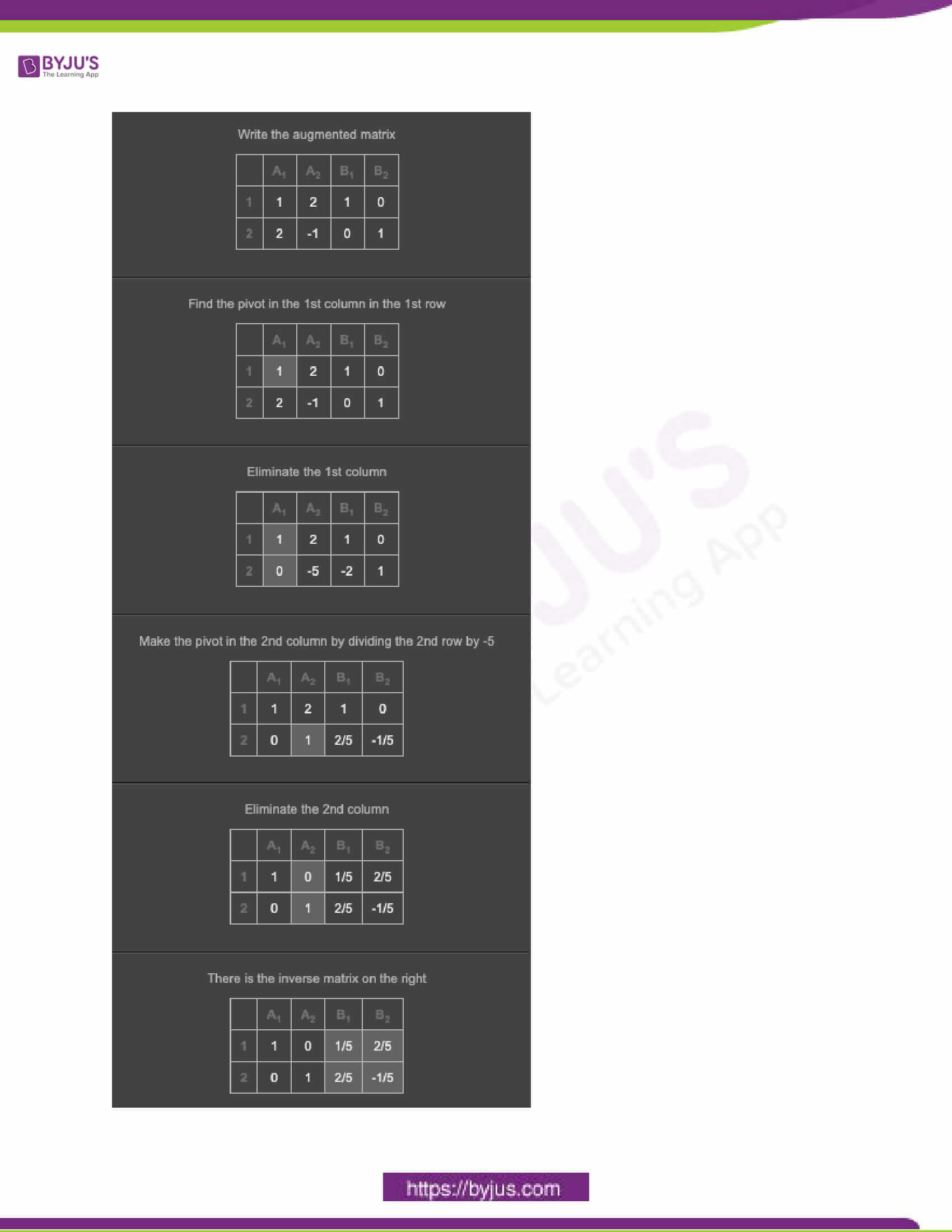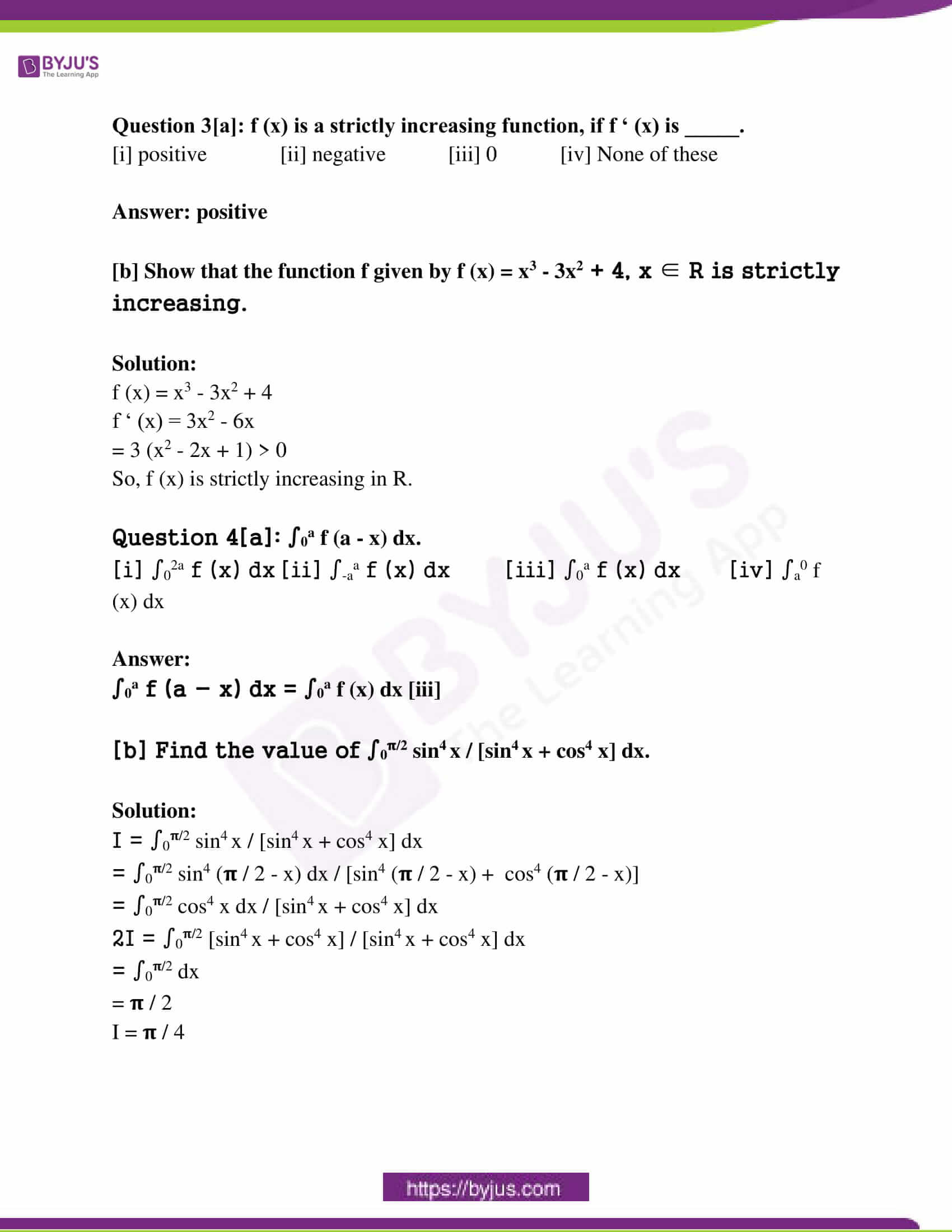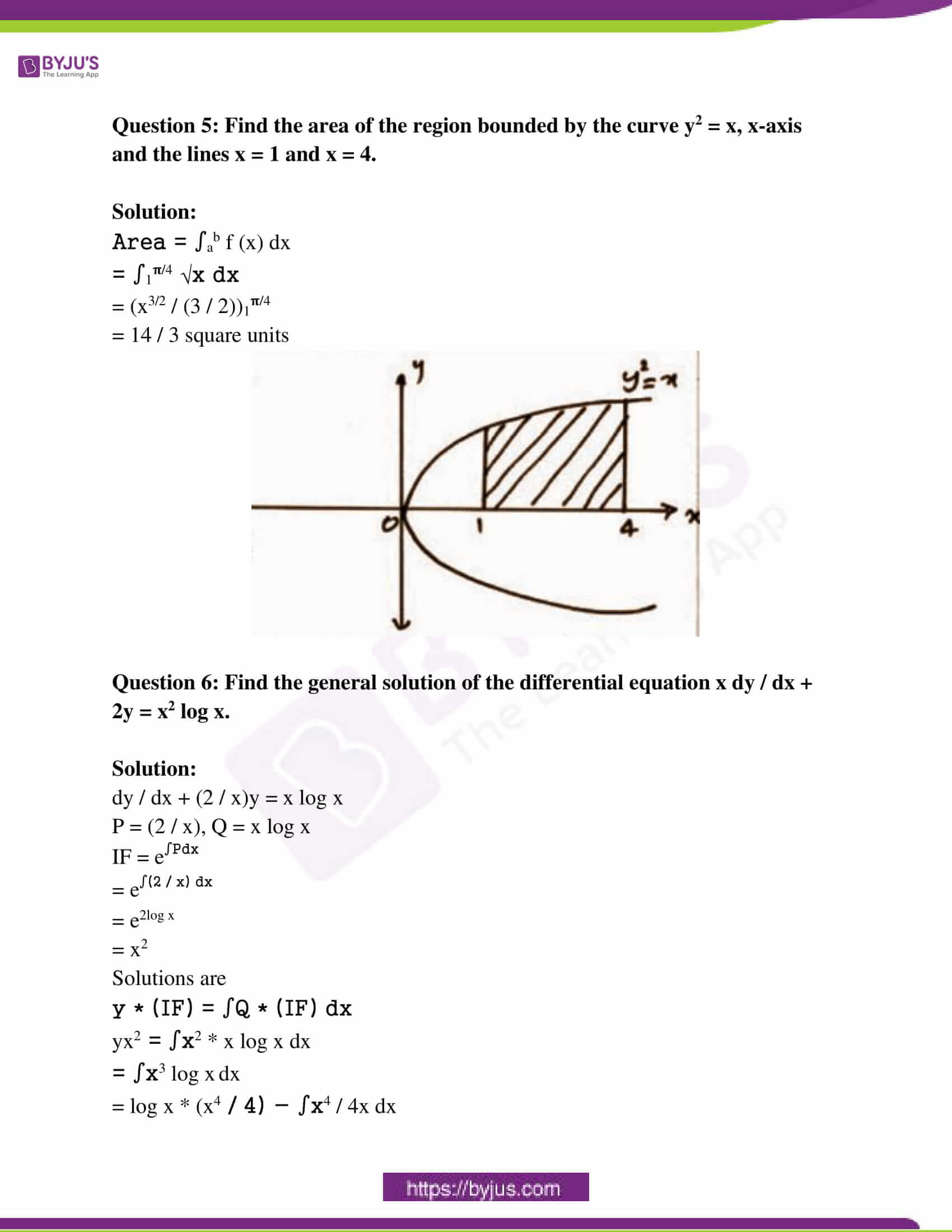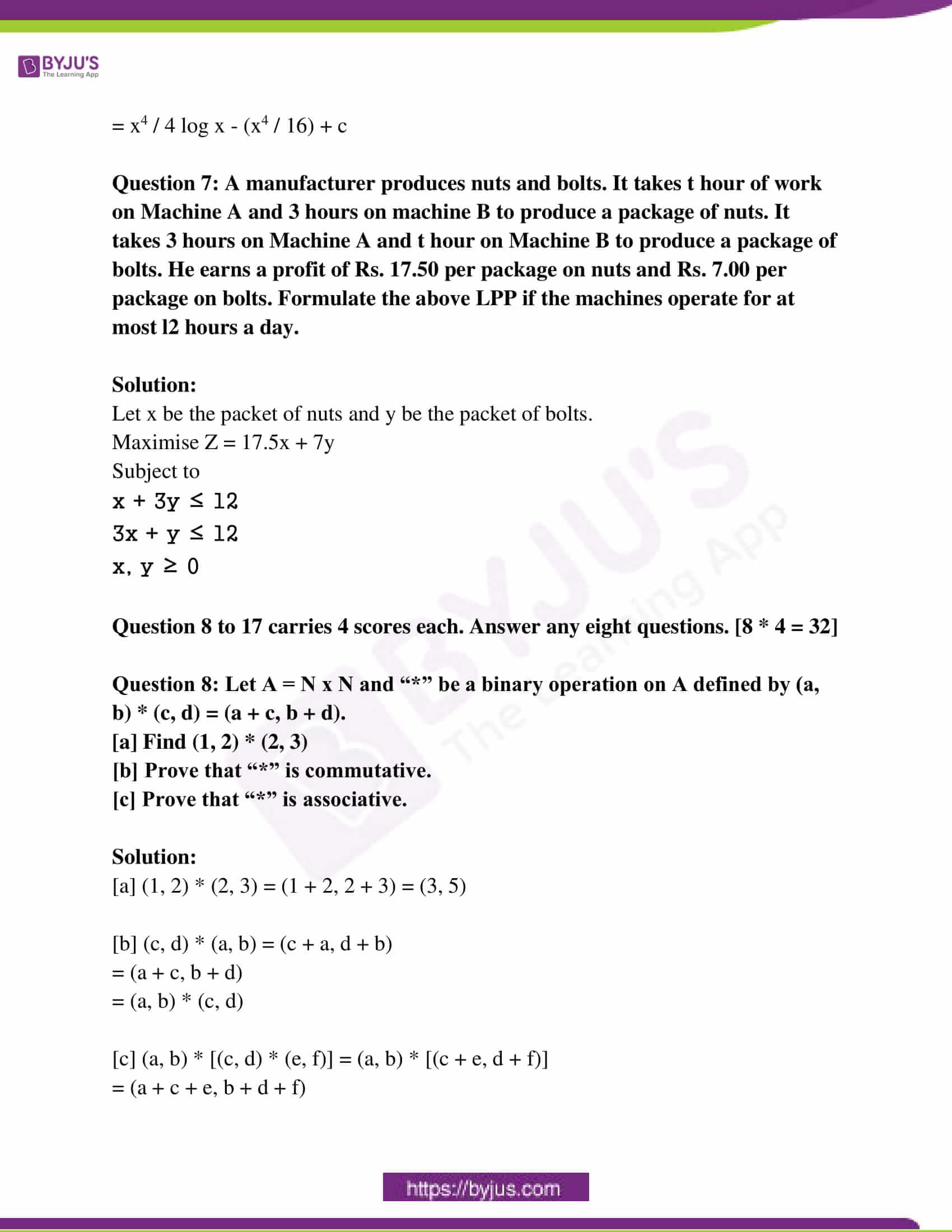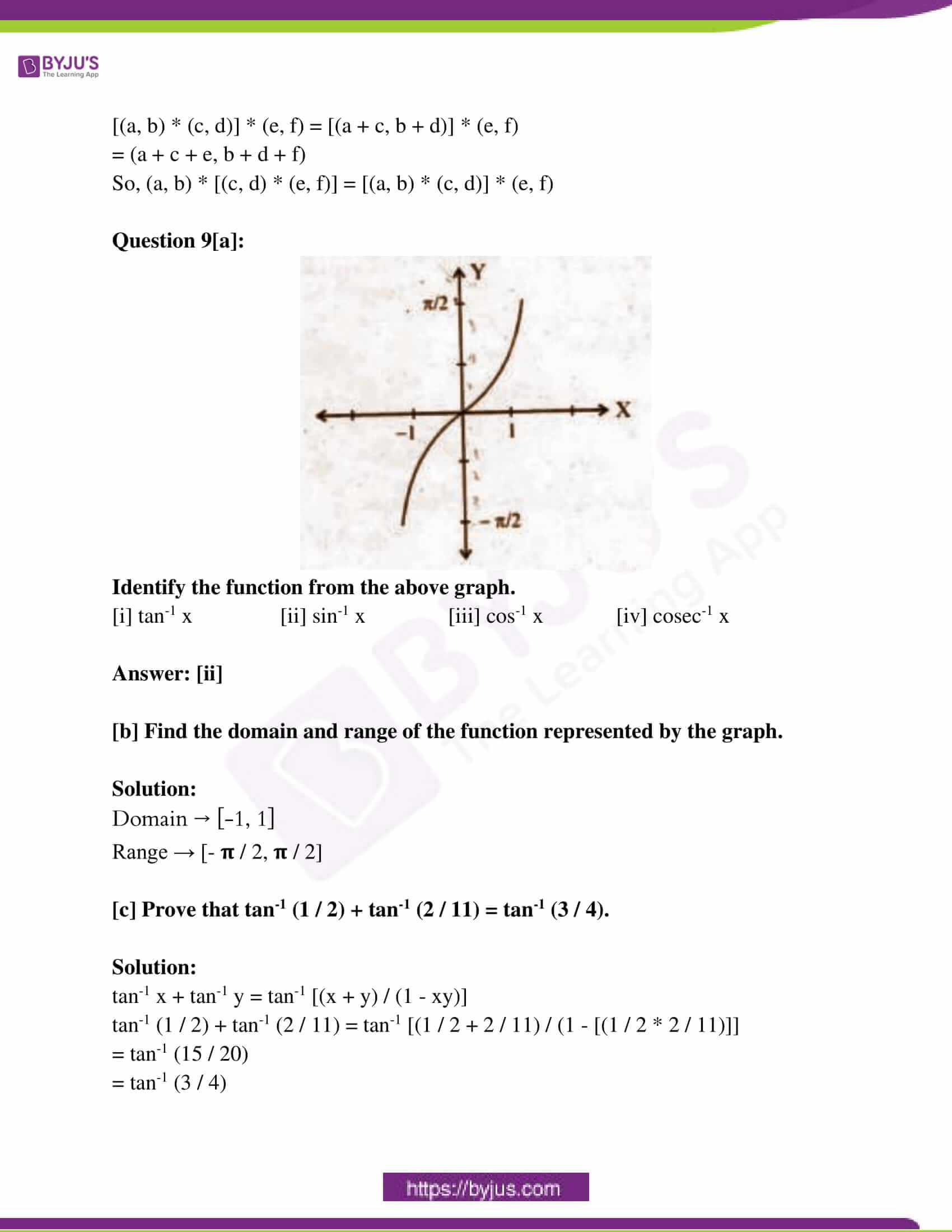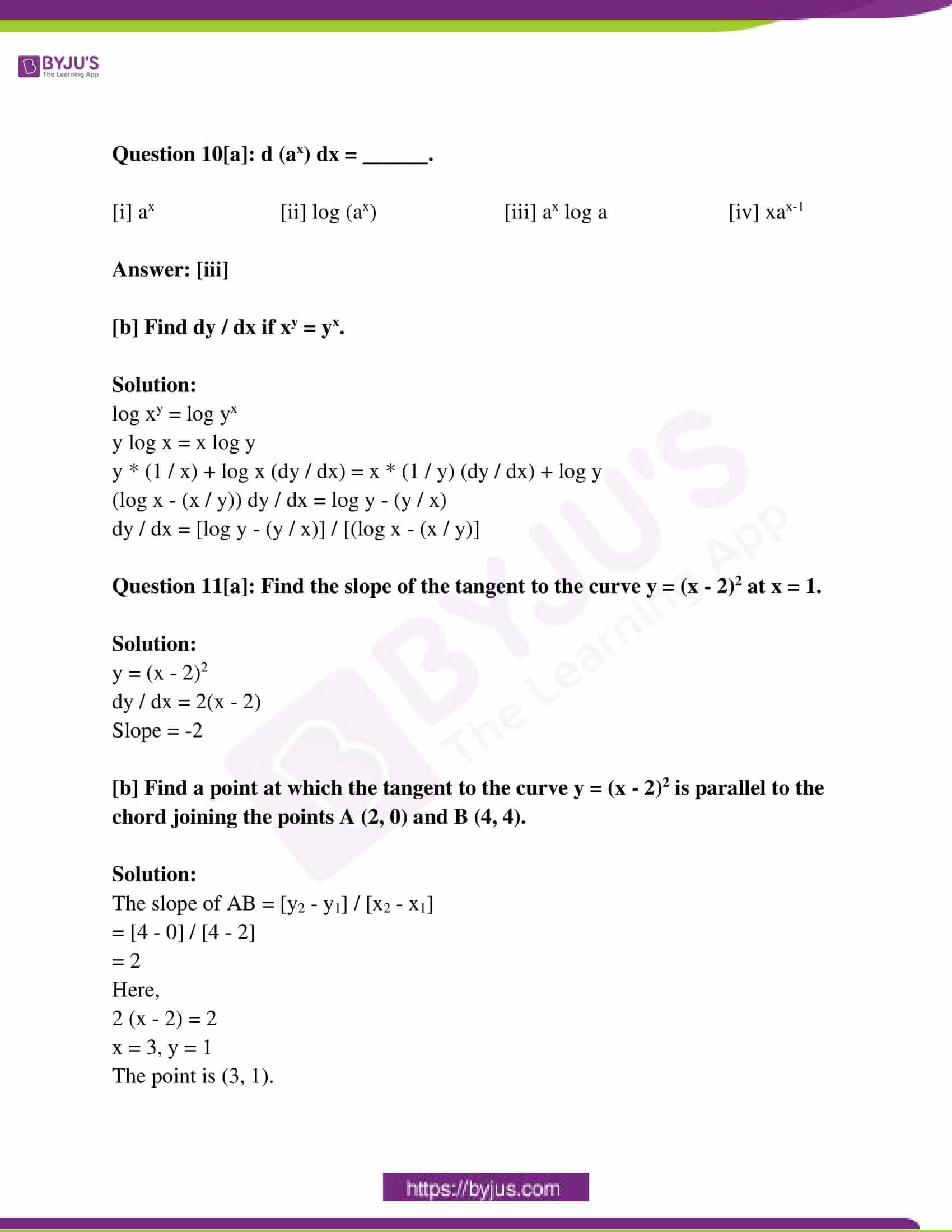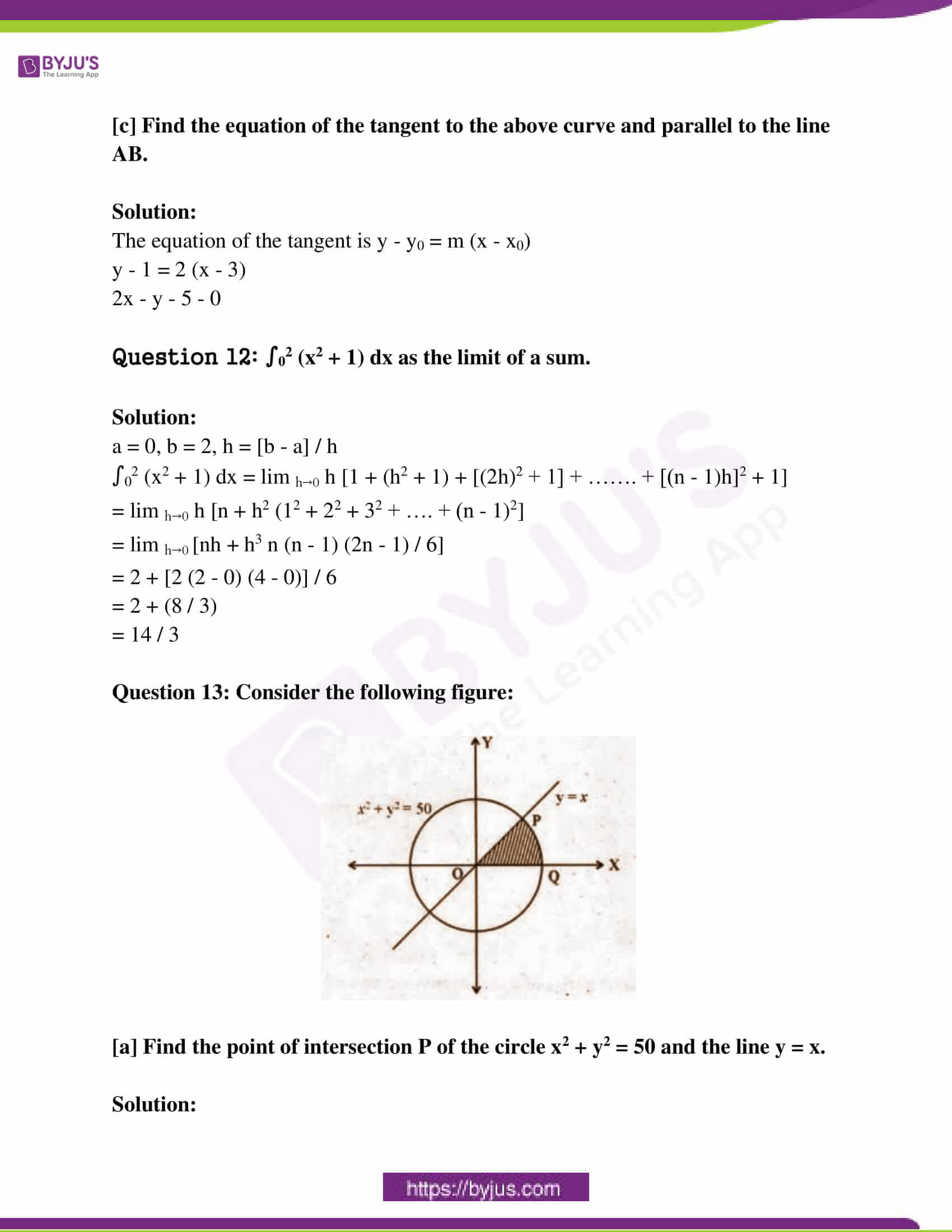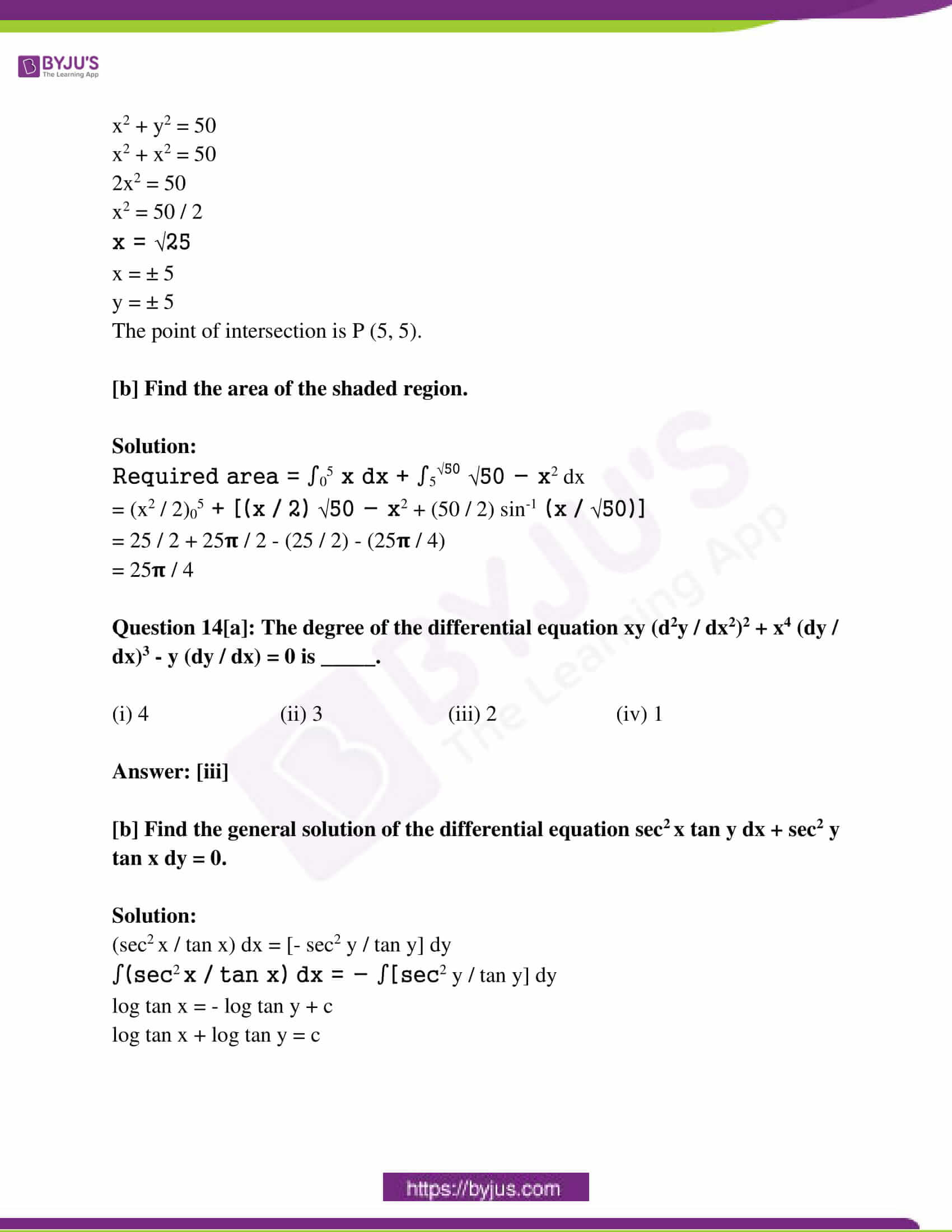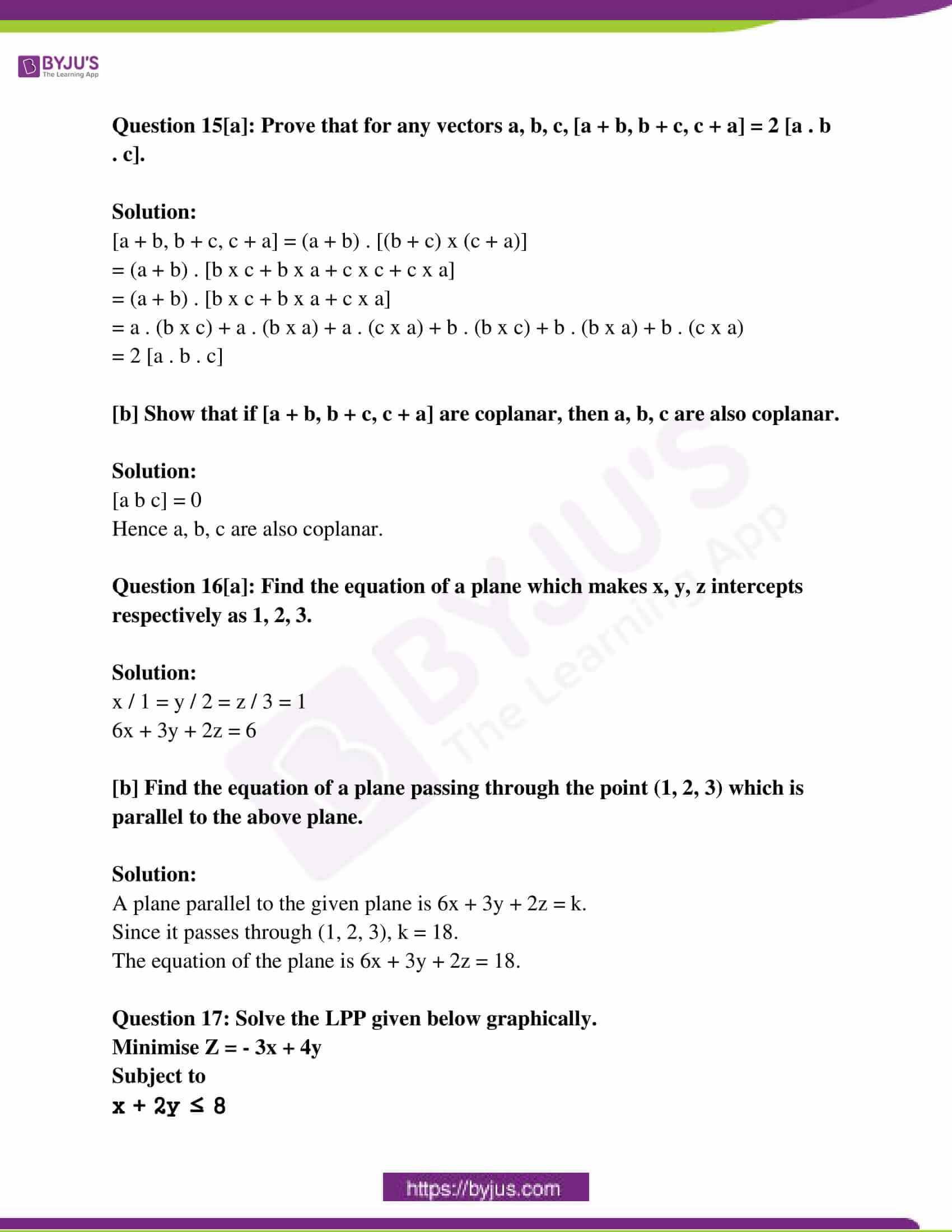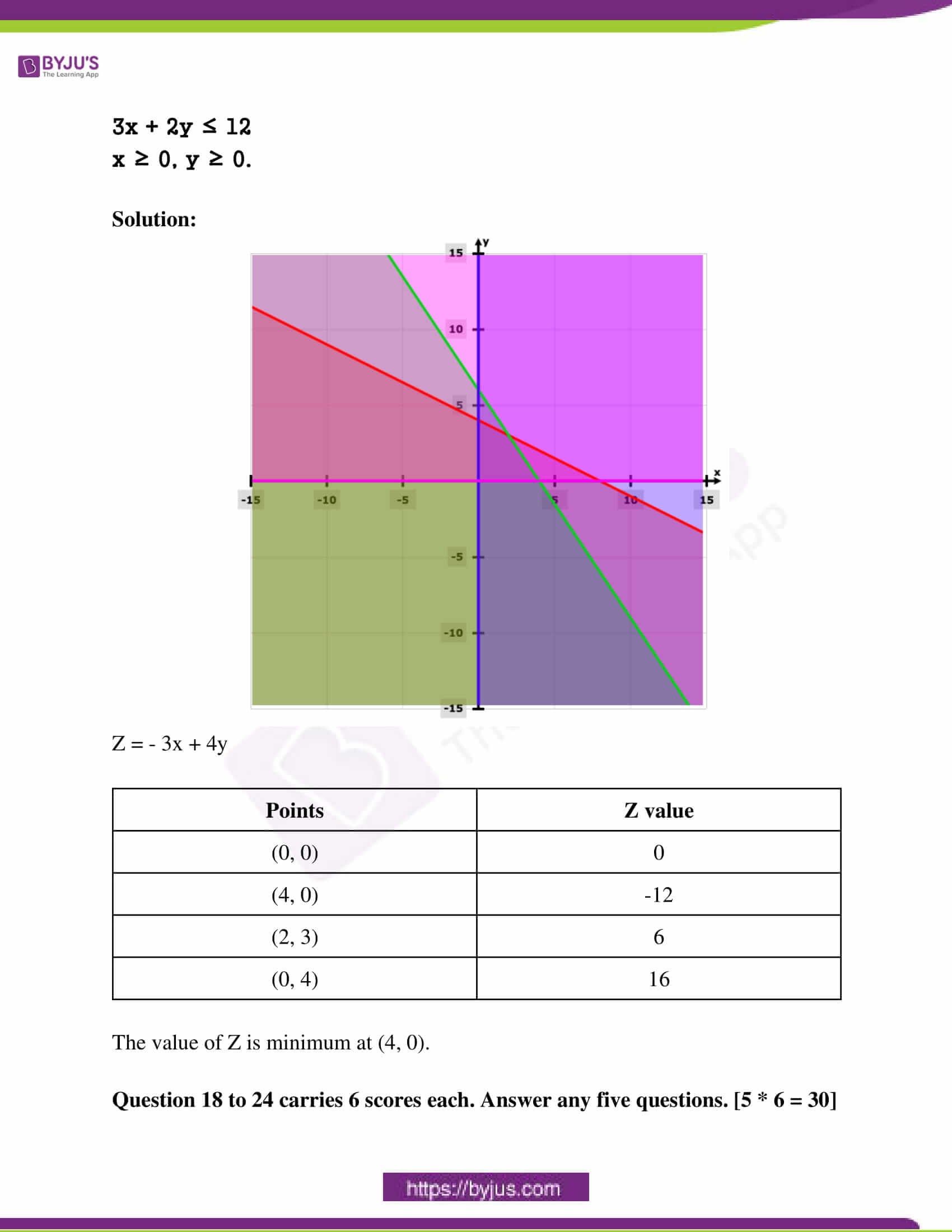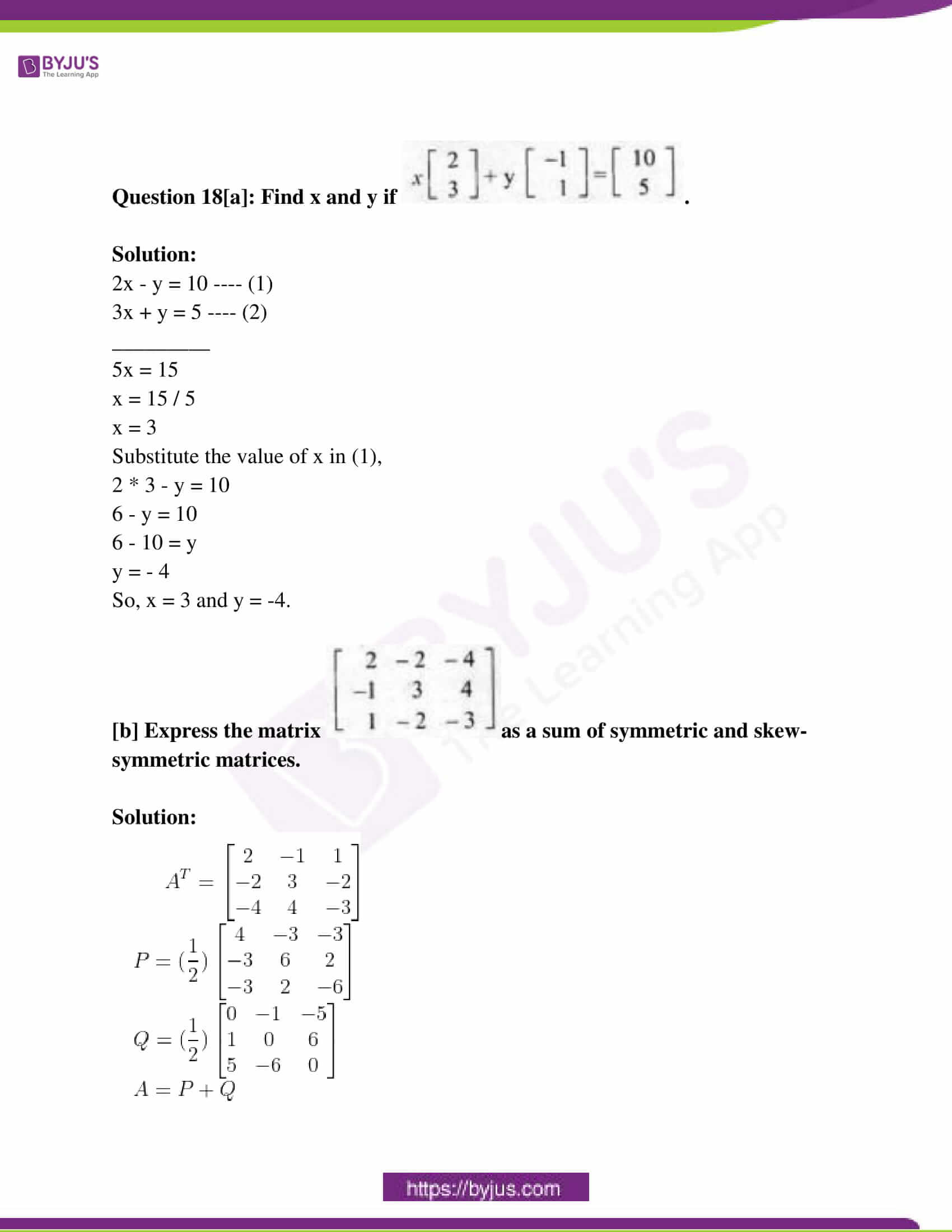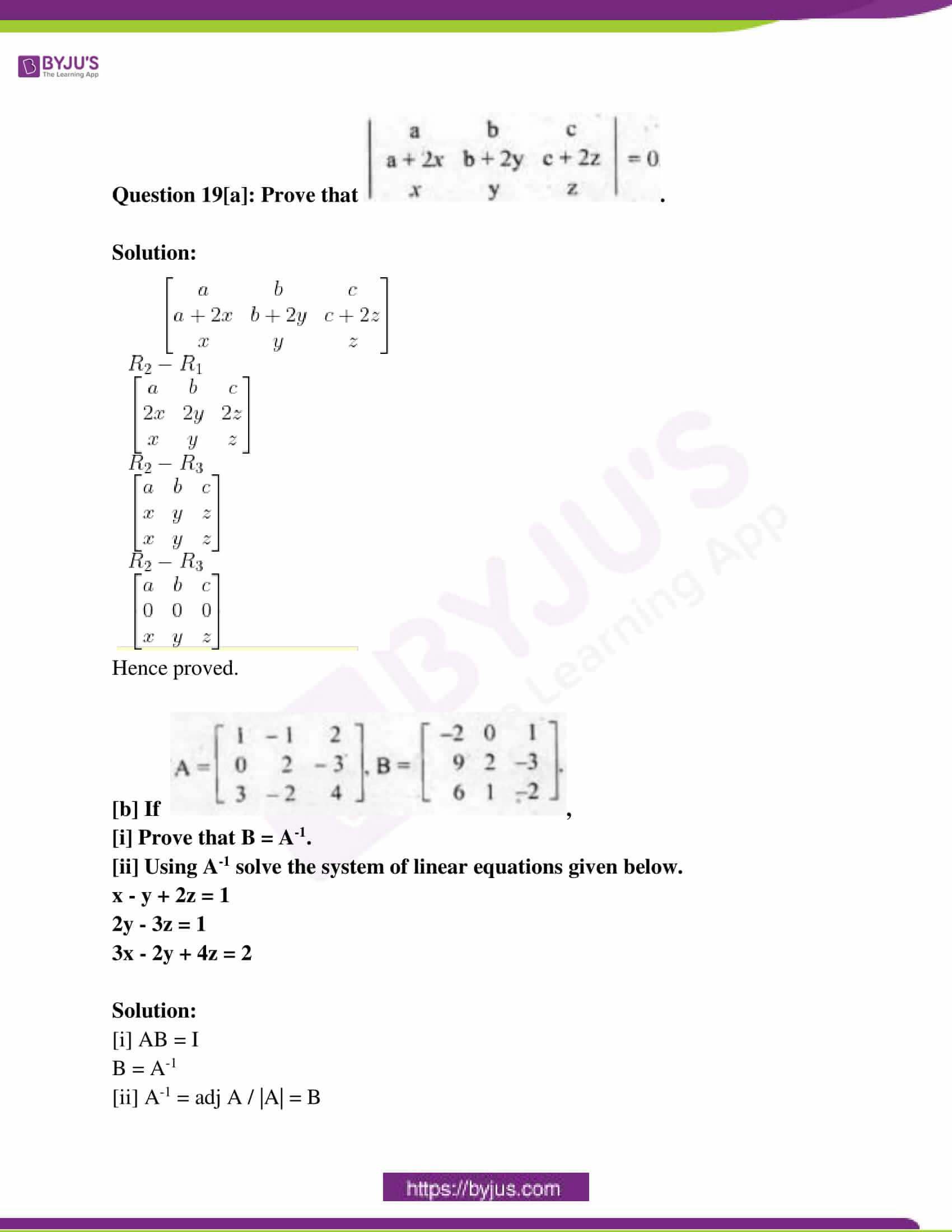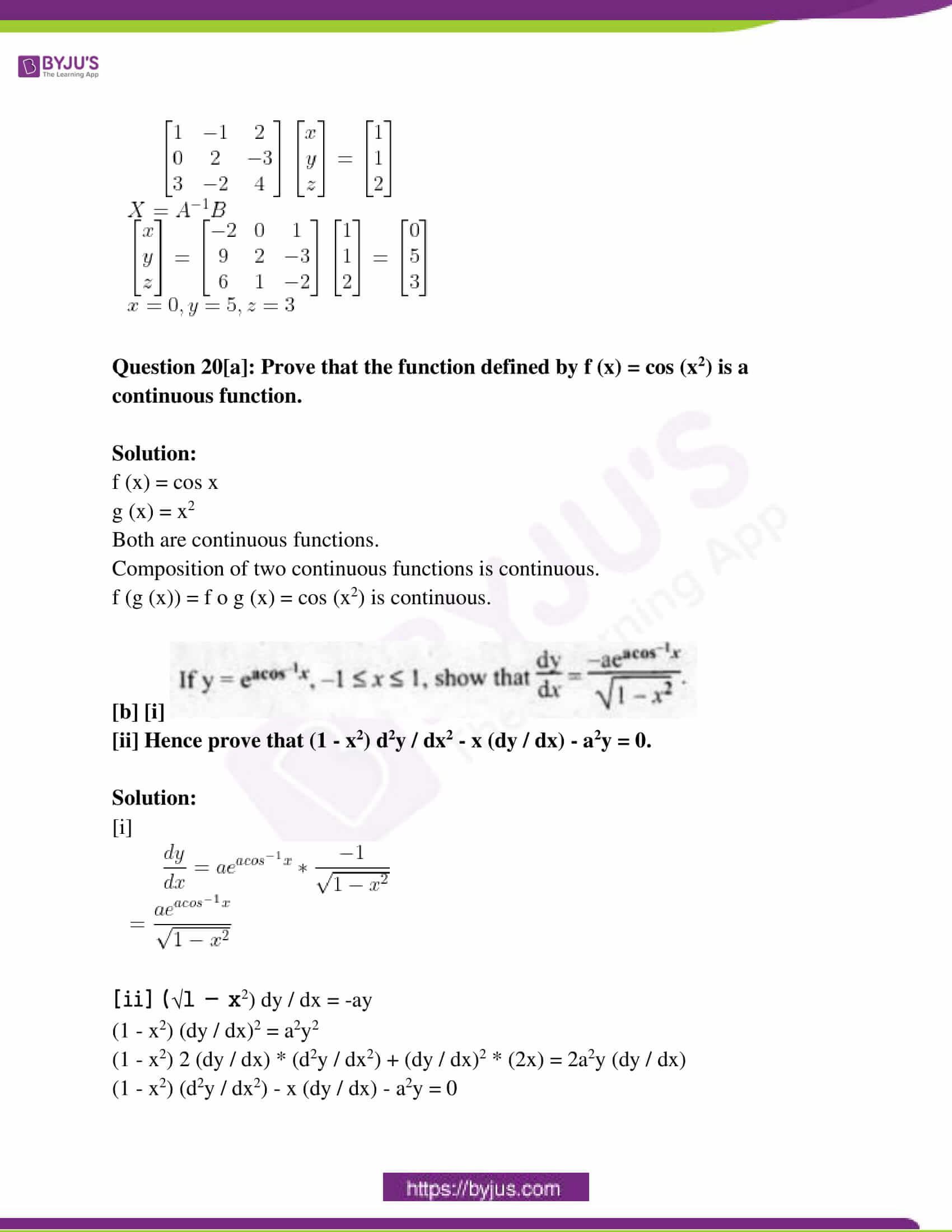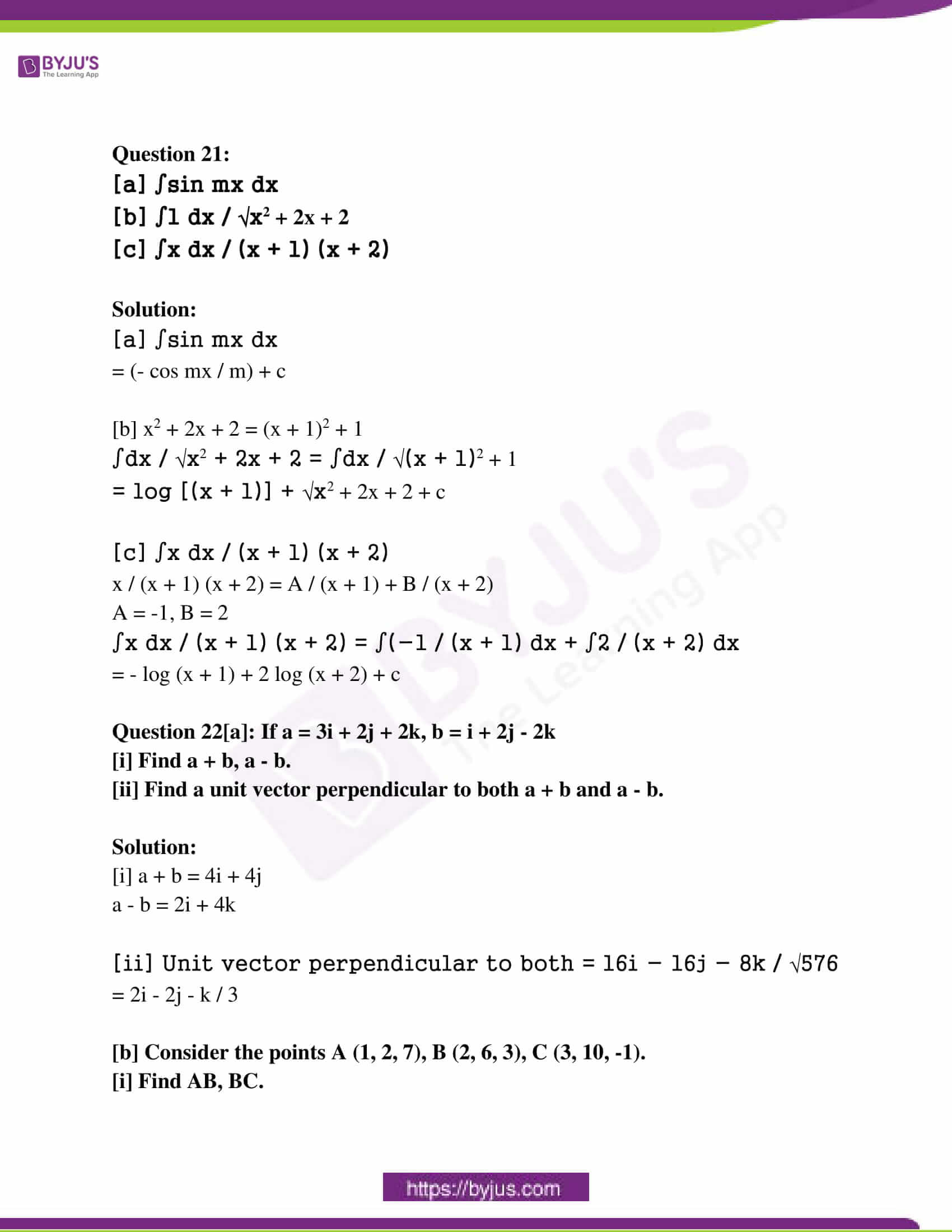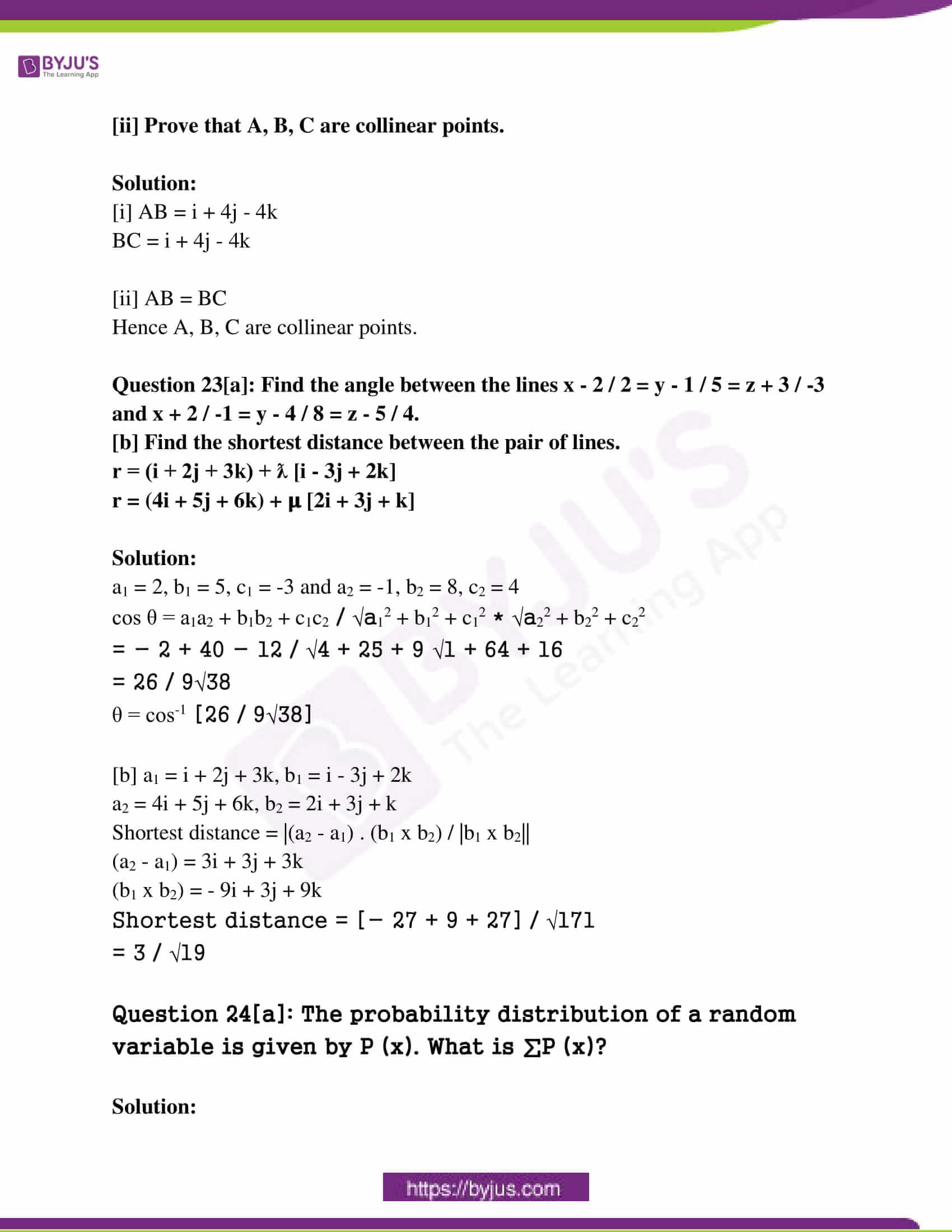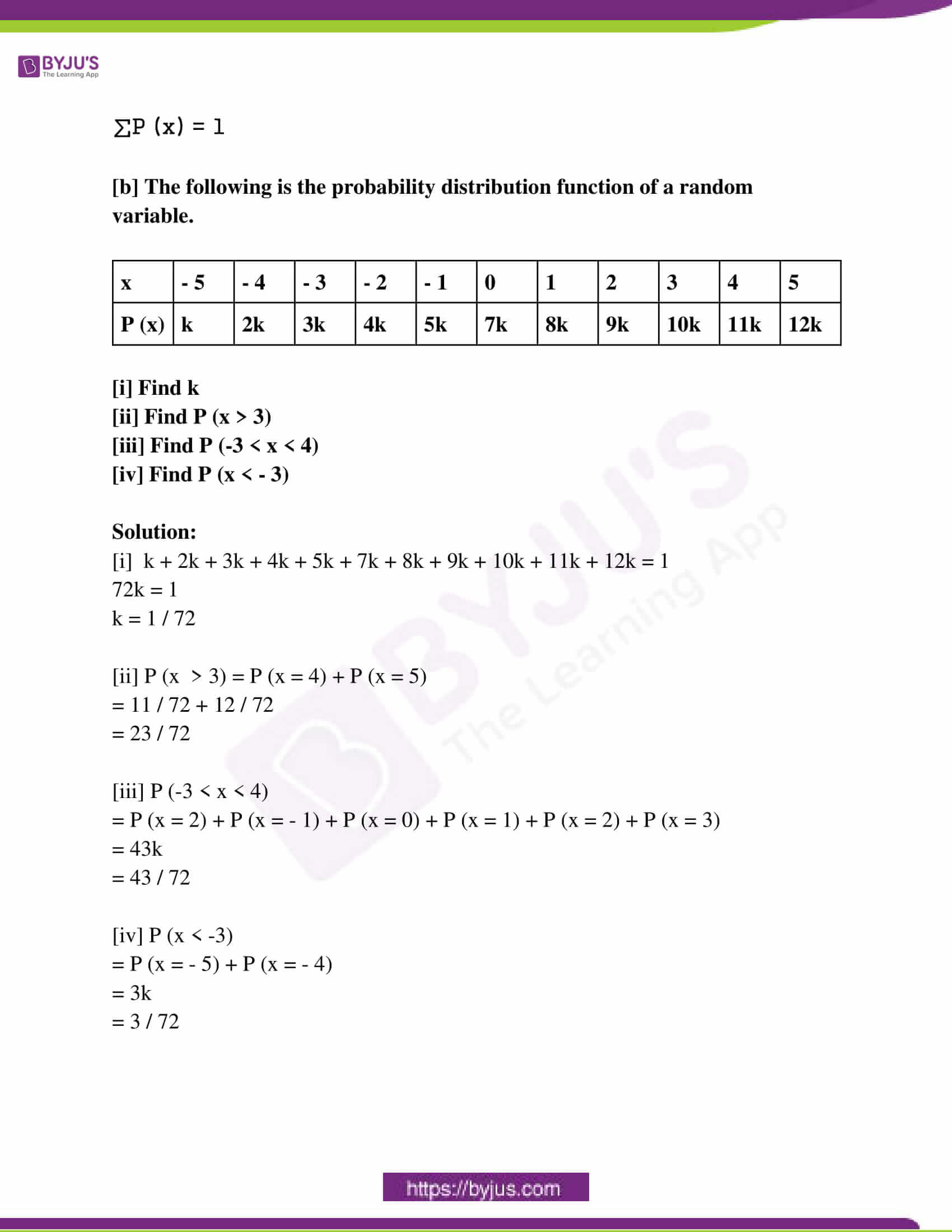Question 1 to 7 carries 3 scores each. Answer any six questions. [6 * 3 = 18]

Question 1: If f (x) = x / (x – 1), x ≠ 1,

[a] Find f o f (x)

[b] Find the inverse of f

Solution:

[a] f o f (x) = f ( f (x))

= f (x / x – 1)

= (x / x – 1) / [(x / x – 1) – 1]

= x / [x – (x – 1)]

= x

[b] y = x / (x – 1)

xy – x = y

xy – y = x

y (x – 1) = x

f -1 (y) = y / (y – 1)

f -1 (x) = x / (x – 1)

Question 2: Using elementary row operations, find the inverse of the matrix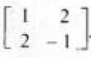.

Solution: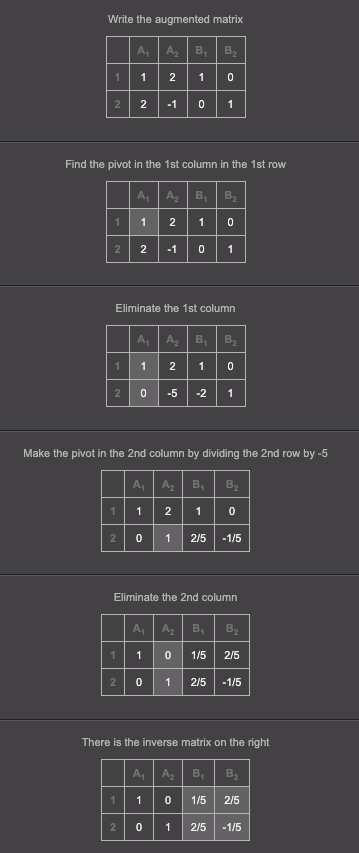Question 3[a]: f (x) is a strictly increasing function, if f ‘ (x) is _____.

[i] positive [ii] negative [iii] 0 [iv] None of these

[b] Show that the function f given by f (x) = x3 – 3x2 + 4, x ∈ R is strictly increasing.

Solution:

f (x) = x3 – 3x2 + 4

f ‘ (x) = 3x2 – 6x

= 3 (x2 – 2x + 1) > 0

So, f (x) is strictly increasing in R.

Question 4[a]: ∫0a f (a – x) dx.

[i] ∫02a f (x) dx [ii] ∫-aa f (x) dx [iii] ∫0a f (x) dx [iv] ∫a0 f (x) dx

0a f (a – x) dx = ∫0a f (x) dx [iii]

[b] Find the value of ∫0𝛑/2 sin4 x / [sin4 x + cos4 x] dx.

Solution:

I = ∫0𝛑/2 sin4 x / [sin4 x + cos4 x] dx

= ∫0𝛑/2 sin4 (𝛑 / 2 – x) dx / [sin4 (𝛑 / 2 – x) + cos4 (𝛑 / 2 – x)]

= ∫0𝛑/2 cos4 x dx / [sin4 x + cos4 x] dx

2I = ∫0𝛑/2 [sin4 x + cos4 x] / [sin4 x + cos4 x] dx

= ∫0𝛑/2 dx

= 𝛑 / 2

I = 𝛑 / 4

Question 5: Find the area of the region bounded by the curve y2 = x, x-axis and the lines x = 1 and x = 4.

Solution:

Area = ∫ab f (x) dx

= ∫1𝛑/4 √x dx

= (x3/2 / (3 / 2))1𝛑/4

= 14 / 3 square units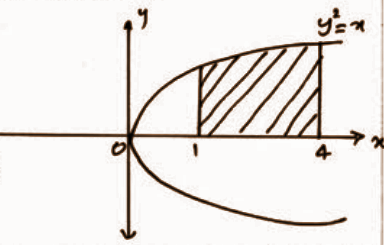Question 6: Find the general solution of the differential equation x dy / dx + 2y = x2 log x.

Solution:

dy / dx + (2 / x)y = x log x

P = (2 / x), Q = x log x

IF = e∫Pdx

= e∫(2 / x) dx

= e2log x

= x2

Solutions are

y * (IF) = ∫Q * (IF) dx

yx2 = ∫x2 * x log x dx

= ∫x3 log x dx

= log x * (x4 / 4) – ∫x4 / 4x dx

= x4 / 4 log x – (x4 / 16) + c

Question 7: A manufacturer produces nuts and bolts. It takes t hour of work on Machine A and 3 hours on machine B to produce a package of nuts. It takes 3 hours on Machine A and t hour on Machine B to produce a package of bolts. He earns a profit of Rs. 17.50 per package on nuts and Rs. 7.00 per package on bolts. Formulate the above LPP if the machines operate for at most l2 hours a day.

Solution:

Let x be the packet of nuts and y be the packet of bolts.

Maximise Z = 17.5x + 7y

Subject to

x + 3y ≤ 12

3x + y ≤ 12

x, y ≥ 0

Question 8 to 17 carries 4 scores each. Answer any eight questions. [8 * 4 = 32]

Question 8: Let A = N x N and “*” be a binary operation on A defined by (a, b) * (c, d) = (a + c, b + d).

[a] Find (1, 2) * (2, 3)

[b] Prove that “*” is commutative.

[c] Prove that “*” is associative.

Solution:

[a] (1, 2) * (2, 3) = (1 + 2, 2 + 3) = (3, 5)

[b] (c, d) * (a, b) = (c + a, d + b)

= (a + c, b + d)

= (a, b) * (c, d)

[c] (a, b) * [(c, d) * (e, f)] = (a, b) * [(c + e, d + f)]

= (a + c + e, b + d + f)

[(a, b) * (c, d)] * (e, f) = [(a + c, b + d)] * (e, f)

= (a + c + e, b + d + f)

So, (a, b) * [(c, d) * (e, f)] = [(a, b) * (c, d)] * (e, f)

Question 9[a]: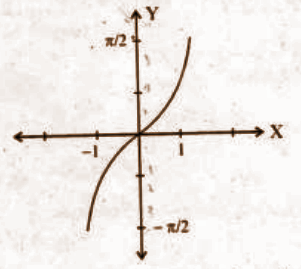Identify the function from the above graph.

[i] tan-1 x [ii] sin-1 x [iii] cos-1 x [iv] cosec-1 x

[b] Find the domain and range of the function represented by the graph.

Solution:

Domain → [-1, 1]

Range → [- 𝛑 / 2, 𝛑 / 2]

[c] Prove that tan-1 (1 / 2) + tan-1 (2 / 11) = tan-1 (3 / 4).

Solution:

tan-1 x + tan-1 y = tan-1 [(x + y) / (1 – xy)]

tan-1 (1 / 2) + tan-1 (2 / 11) = tan-1 [(1 / 2 + 2 / 11) / (1 – [(1 / 2 * 2 / 11)]]

= tan-1 (15 / 20)

= tan-1 (3 / 4)

Question 10[a]: d (ax) dx = ______.

[i] ax [ii] log (ax) [iii] ax log a [iv] xax-1

[b] Find dy / dx if xy = yx.

Solution:

log xy = log yx

y log x = x log y

y * (1 / x) + log x (dy / dx) = x * (1 / y) (dy / dx) + log y

(log x – (x / y)) dy / dx = log y – (y / x)

dy / dx = [log y – (y / x)] / [(log x – (x / y)]

Question 11[a]: Find the slope of the tangent to the curve y = (x – 2)2 at x = 1.

Solution:

y = (x – 2)2

dy / dx = 2(x – 2)

Slope = -2

[b] Find a point at which the tangent to the curve y = (x – 2)2 is parallel to the chord joining the points A (2, 0) and B (4, 4).

Solution:

The slope of AB = [y2 – y1] / [x2 – x1]

= [4 – 0] / [4 – 2]

= 2

Here,

2 (x – 2) = 2

x = 3, y = 1

The point is (3, 1).

[c] Find the equation of the tangent to the above curve and parallel to the line AB.

Solution:

The equation of the tangent is y – y0 = m (x – x0)

y – 1 = 2 (x – 3)

2x – y – 5 – 0

Question 12: ∫02 (x2 + 1) dx as the limit of a sum.

Solution:

a = 0, b = 2, h = [b – a] / h

02 (x2 + 1) dx = lim h→0 h [1 + (h2 + 1) + [(2h)2 + 1] + ……. + [(n – 1)h]2 + 1]

= lim h→0 h [n + h2 (12 + 22 + 32 + …. + (n – 1)2]

= lim h→0 [nh + h3 n (n – 1) (2n – 1) / 6]

= 2 + [2 (2 – 0) (4 – 0)] / 6

= 2 + (8 / 3)

= 14 / 3

Question 13: Consider the following figure: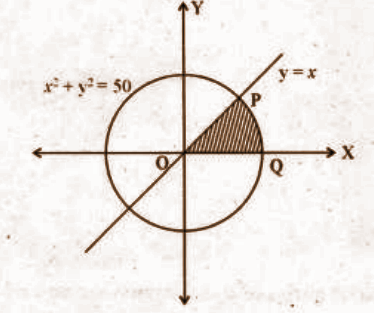[a] Find the point of intersection P of the circle x2 + y2 = 50 and the line y = x.

Solution:

x2 + y2 = 50

x2 + x2 = 50

2x2 = 50

x2 = 50 / 2

x = √25

x = ± 5

y = ± 5

The point of intersection is P (5, 5).

[b] Find the area of the shaded region.

Solution:

Required area = ∫05 x dx + ∫5√50 √50 – x2 dx

= (x2 / 2)05 + [(x / 2) √50 – x2 + (50 / 2) sin-1 (x / √50)]

= 25 / 2 + 25𝛑 / 2 – (25 / 2) – (25𝛑 / 4)

= 25𝛑 / 4

Question 14[a]: The degree of the differential equation xy (d2y / dx2)2 + x4 (dy / dx)3 – y (dy / dx) = 0 is _____.

(i) 4 (ii) 3 (iii) 2 (iv) 1

[b] Find the general solution of the differential equation sec2 x tan y dx + sec2 y tan x dy = 0.

Solution:

(sec2 x / tan x) dx = [- sec2 y / tan y] dy

∫(sec2 x / tan x) dx = – ∫[sec2 y / tan y] dy

log tan x = – log tan y + c

log tan x + log tan y = c

Question 15[a]: Prove that for any vectors a, b, c, [a + b, b + c, c + a] = 2 [a . b . c].

Solution:

[a + b, b + c, c + a] = (a + b) . [(b + c) x (c + a)]

= (a + b) . [b x c + b x a + c x c + c x a]

= (a + b) . [b x c + b x a + c x a]

= a . (b x c) + a . (b x a) + a . (c x a) + b . (b x c) + b . (b x a) + b . (c x a)

= 2 [a . b . c]

[b] Show that if [a + b, b + c, c + a] are coplanar, then a, b, c are also coplanar.

Solution:

[a b c] = 0

Hence a, b, c are also coplanar.

Question 16[a]: Find the equation of a plane which makes x, y, z intercepts respectively as 1, 2, 3.

Solution:

x / 1 = y / 2 = z / 3 = 1

6x + 3y + 2z = 6

[b] Find the equation of a plane passing through the point (1, 2, 3) which is parallel to the above plane.

Solution:

A plane parallel to the given plane is 6x + 3y + 2z = k.

Since it passes through (1, 2, 3), k = 18.

The equation of the plane is 6x + 3y + 2z = 18.

Question 17: Solve the LPP given below graphically.

Minimise Z = – 3x + 4y

Subject to

x + 2y ≤ 8

3x + 2y ≤ 12

x ≥ 0, y ≥ 0.

Solution: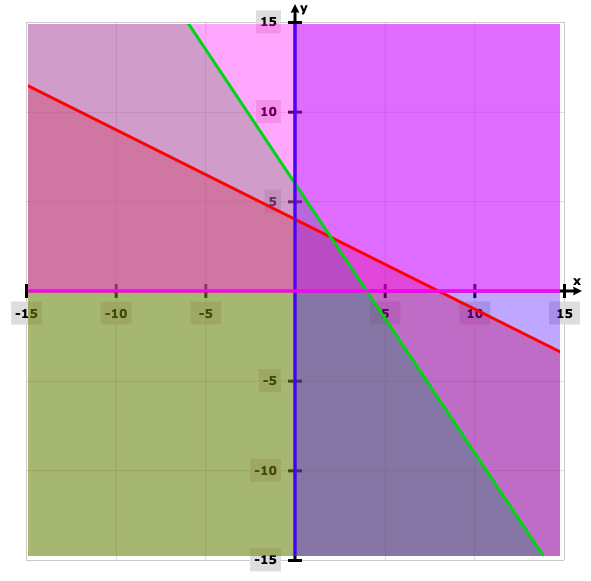Z = – 3x + 4y

 Points Z value (0, 0) 0 (4, 0) -12 (2, 3) 6 (0, 4) 16

The value of Z is minimum at (4, 0).

Question 18 to 24 carries 6 scores each. Answer any five questions. [5 * 6 = 30]

Question 18[a]: Find x and y if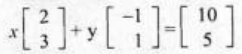.

Solution:

2x – y = 10 —- (1)

3x + y = 5 —- (2)

_________

5x = 15

x = 15 / 5

x = 3

Substitute the value of x in (1),

2 * 3 – y = 10

6 – y = 10

6 – 10 = y

y = – 4

So, x = 3 and y = -4.

[b] Express the matrix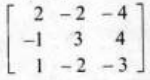as a sum of symmetric and skew-symmetric matrices.

Solution: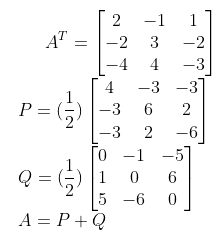Question 19[a]: Prove that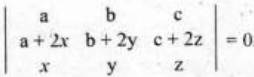.

Solution: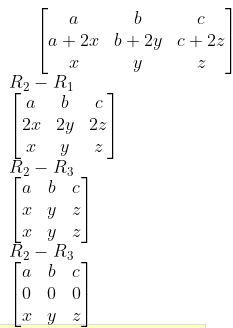Hence proved.

[b] If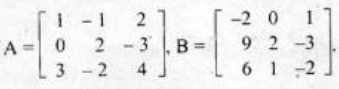,

[i] Prove that B = A-1.

[ii] Using A-1 solve the system of linear equations given below.

x – y + 2z = 1

2y – 3z = 1

3x – 2y + 4z = 2

Solution:

[i] AB = I

B = A-1

[ii] A-1 = adj A / |A| = B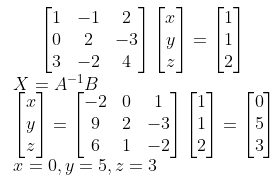Question 20[a]: Prove that the function defined by f (x) = cos (x2) is a continuous function.

Solution:

f (x) = cos x

g (x) = x2

Both are continuous functions.

Composition of two continuous functions is continuous.

f (g (x)) = f o g (x) = cos (x2) is continuous.

[b] [i]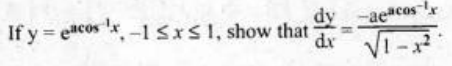[ii] Hence prove that (1 – x2) d2y / dx2 – x (dy / dx) – a2y = 0.

Solution:

[i]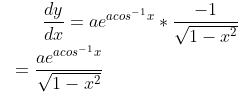[ii] (√1 – x2) dy / dx = -ay

(1 – x2) (dy / dx)2 = a2y2

(1 – x2) 2 (dy / dx) * (d2y / dx2) + (dy / dx)2 * (2x) = 2a2y (dy / dx)

(1 – x2) (d2y / dx2) – x (dy / dx) – a2y = 0

Question 21:

[a] ∫sin mx dx

[b] ∫1 dx / √x2 + 2x + 2

[c] ∫x dx / (x + 1) (x + 2)

Solution:

[a] ∫sin mx dx

= (- cos mx / m) + c

[b] x2 + 2x + 2 = (x + 1)2 + 1

∫dx / √x2 + 2x + 2 = ∫dx / √(x + 1)2 + 1

= log [(x + 1)] + √x2 + 2x + 2 + c

[c] ∫x dx / (x + 1) (x + 2)

x / (x + 1) (x + 2) = A / (x + 1) + B / (x + 2)

A = -1, B = 2

∫x dx / (x + 1) (x + 2) = ∫(-1 / (x + 1) dx + ∫2 / (x + 2) dx

= – log (x + 1) + 2 log (x + 2) + c

Question 22[a]: If a = 3i + 2j + 2k, b = i + 2j – 2k

[i] Find a + b, a – b.

[ii] Find a unit vector perpendicular to both a + b and a – b.

Solution:

[i] a + b = 4i + 4j

a – b = 2i + 4k

[ii] Unit vector perpendicular to both = 16i – 16j – 8k / √576

= 2i – 2j – k / 3

[b] Consider the points A (1, 2, 7), B (2, 6, 3), C (3, 10, -1).

[i] Find AB, BC.

[ii] Prove that A, B, C are collinear points.

Solution:

[i] AB = i + 4j – 4k

BC = i + 4j – 4k

[ii] AB = BC

Hence A, B, C are collinear points.

Question 23[a]: Find the angle between the lines x – 2 / 2 = y – 1 / 5 = z + 3 / -3 and x + 2 / -1 = y – 4 / 8 = z – 5 / 4.

[b] Find the shortest distance between the pair of lines.

r = (i + 2j + 3k) + ƛ [i – 3j + 2k]

r = (4i + 5j + 6k) + 𝛍 [2i + 3j + k]

Solution:

a1 = 2, b1 = 5, c1 = -3 and a2 = -1, b2 = 8, c2 = 4

cos θ = a1a2 + b1b2 + c1c2 / √a12 + b12 + c12 * √a22 + b22 + c22

= – 2 + 40 – 12 / √4 + 25 + 9 √1 + 64 + 16

= 26 / 9√38

θ = cos-1 [26 / 9√38] [b] a1 = i + 2j + 3k, b1 = i – 3j + 2k

a2 = 4i + 5j + 6k, b2 = 2i + 3j + k

Shortest distance = |(a2 – a1) . (b1 x b2) / |b1 x b2||

(a2 – a1) = 3i + 3j + 3k

(b1 x b2) = – 9i + 3j + 9k

Shortest distance = [- 27 + 9 + 27] / √171

= 3 / √19

Question 24[a]: The probability distribution of a random variable is given by P (x). What is ∑P (x)?

Solution:

∑P (x) = 1

[b] The following is the probability distribution function of a random variable.

 x – 5 – 4 – 3 – 2 – 1 0 1 2 3 4 5 P (x) k 2k 3k 4k 5k 7k 8k 9k 10k 11k 12k

[i] Find k

[ii] Find P (x > 3)

[iii] Find P (-3 < x < 4)

[iv] Find P (x < – 3)

Solution:

[i] k + 2k + 3k + 4k + 5k + 7k + 8k + 9k + 10k + 11k + 12k = 1

72k = 1

k = 1 / 72

[ii] P (x > 3) = P (x = 4) + P (x = 5)

= 11 / 72 + 12 / 72

= 23 / 72

[iii] P (-3 < x < 4)

= P (x = 2) + P (x = – 1) + P (x = 0) + P (x = 1) + P (x = 2) + P (x = 3)

= 43k

= 43 / 72

[iv] P (x < -3)

= P (x = – 5) + P (x = – 4)

= 3k

= 3 / 72